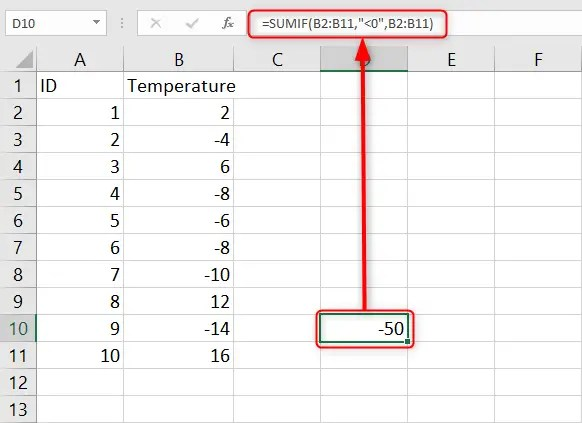# How to sum negative numbers in Excel

When it comes to Excel’s computation, it is an easier task to perform, as the program provides in-built formulas that make your work easier. Knowing how to apply Excel’s formulas helps you in presenting work that is efficient and reliable. One of the most common computations to do in Excel is additions using the SUM function. You can sum negative and positive values in Excel using the formulas that are already in Excel.

In our article below, we explain how to sum only negative numbers in Excel.

## Using the SUMIF Function

Excel’s SUMIF function helps you add only negative or positive values. This formula comes in handy, especially when you want to add only negative numbers in a mix of both positive and negative numbers. You will use the formula:

`=SUMIF (range, criteria, [sum_range])`

Whereby;

Range – represents the criteria range of cells that contain the data you want the function to work on.

Criteria – represents the condition you want to be satisfied to include a cell in the sum. Any operator should be used within double quotes.

Sum_range – represents the operational parameter.

Note:

The SUMIF formula offers an additional advantage as you can add one or more conditions after the comma.

To use the formula:

1. In an open Excel worksheet, click on the Cell where you want your final results displayed.

2. In this cell, type in your formula starting with the equal sign. Here is how your formula will be: =SUMIF(

3. Select the range of cells or values you want to find.

4. You will notice the selected range of cells will appear after the open bracket in your formula.

5. Insert a comma after the range of cells inserted followed by the condition to be met. Your condition should be in double quotes. When looking to sum negative values, you will use “<0” as your condition.

6. Insert a closing bracket after the condition if you have no other conditions to include.As you can see from the image above, the SUMIF function only adds negative numbers. You can change “<0” to “>0” to add only positive numbers in Excel.

## Method 2:

1. In an open Excel workbook, click on the letter at the top of a column counting the negative numbers to highlight it. You can also do this if it is a row that contains negative values that you need to sum.

2. Right-click anywhere within your highlighted cells to display a context menu list.

3. In this list, click on the option Format Cells to display a window.

4. Click on the Number

5. Under the Category section on the left side of the window, click on Number if your cells contain non-currency numerical data. Click on the option Currency if the cells contain currency values. Choose the appearance you want to use for negative values in the Negative section and click the OK6. Indicate the number of decimal points Excel should display in the Decimal Places

7. Select the checkbox next to Use 1,000 separator if you want Excel to insert a comma between every three digits for any values over a thousand.

8. Click the OK

9. Click on an empty cell where you want your results displayed.

10. Click on the equal sign (=) and enter your calculations by referencing the cells with the negative values. Type a minus before a number to indicate that it is negative.

11. Go to the Home tab > Editing group > Sum > AutoSum.

12. Press the Enter key for Excel to calculate all of the data in these cells.